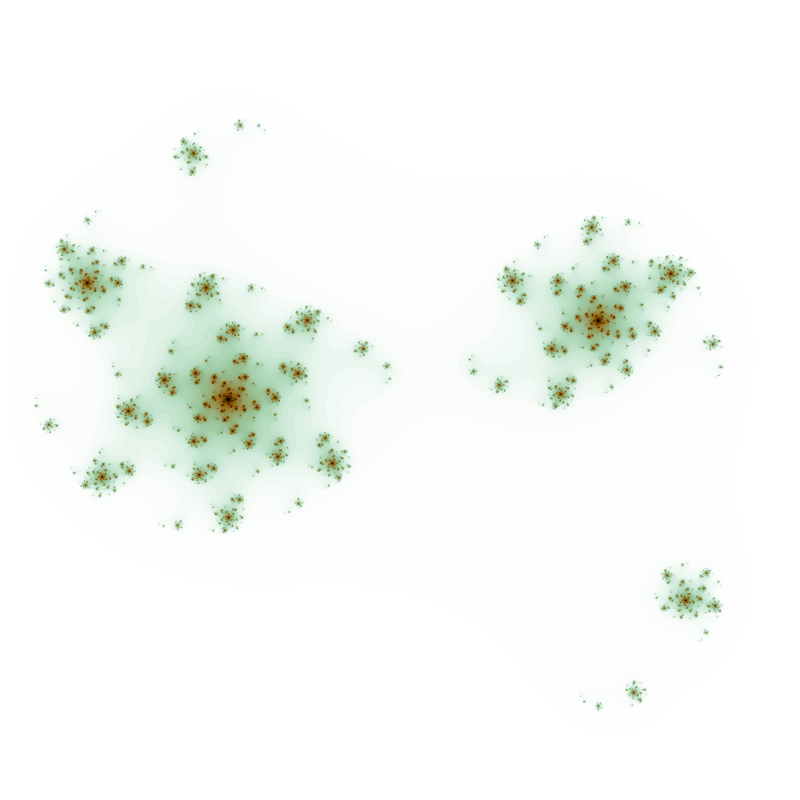# Phoenix Julia

Written by Paul Bourke
April 2022

The so called "phoenix Julia" set is defined the same as the standard julia set except that an additional weighted historical term is added as shown in the equation below. Both "c" and "k" are complex constants.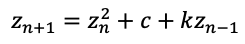First report by Shigehiro Ushiki ans published in 1998 in a paper published in the IEEE Transactions on Circuits and Systems journal. The parameters in that paper were c = 0.5667 and k = -0.5.

c = -0.4 + 0.1i, k = 0.2955c = 0.4, k = -0.25c = 0.566, k = -0.5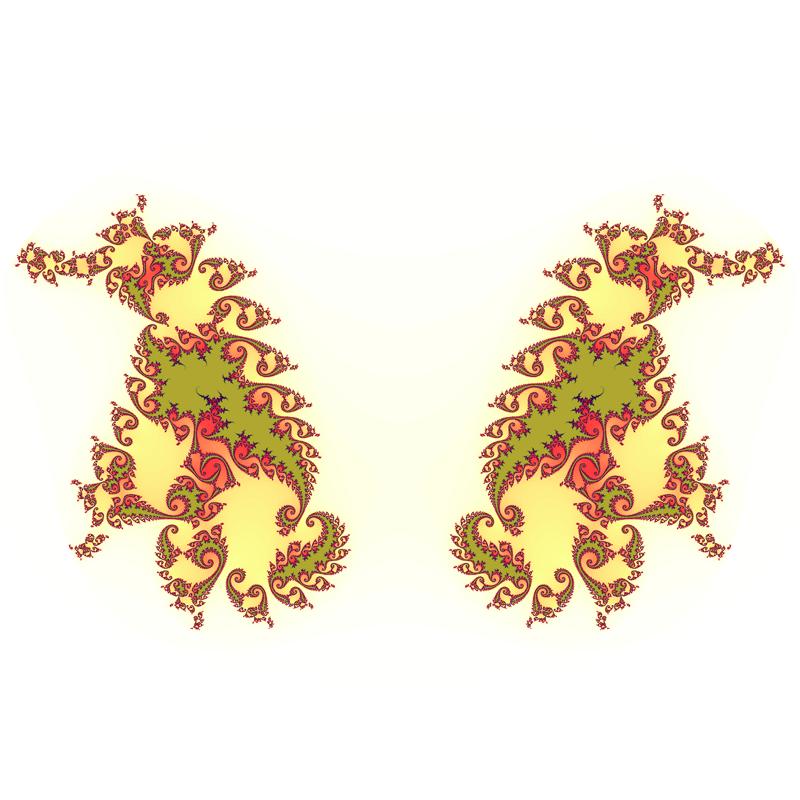c = 0.1 + 0.6i, k = -0.35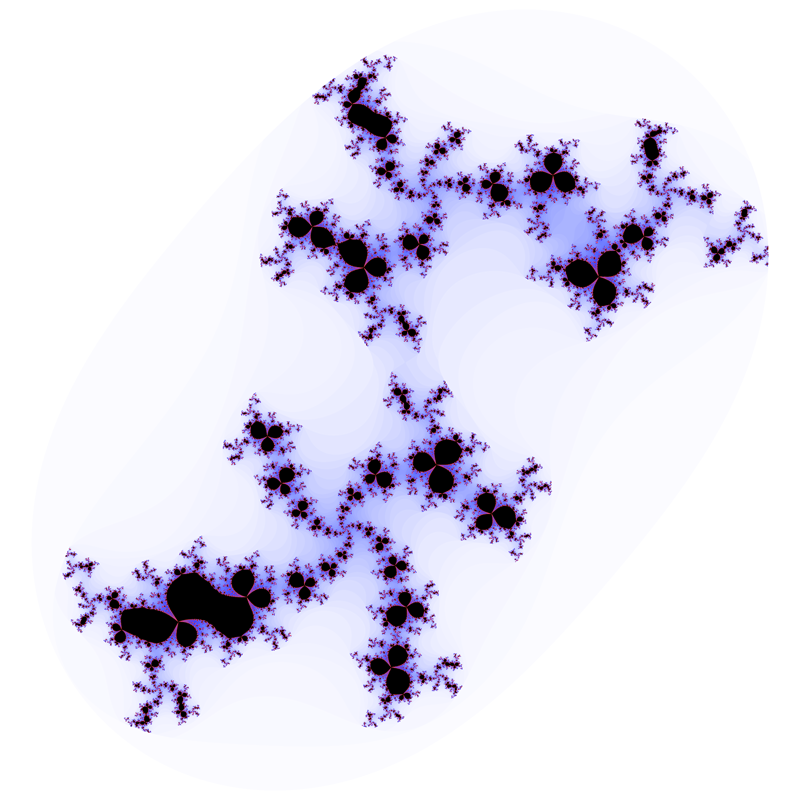c = 0.6 + -0.1i, k = -0.8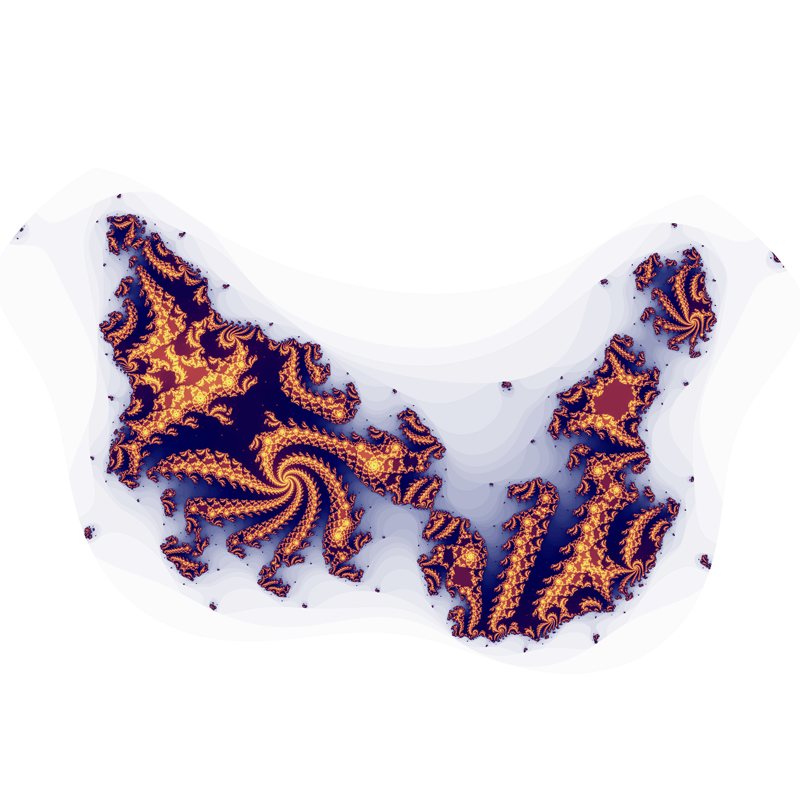c = 0.269, k = -0.01i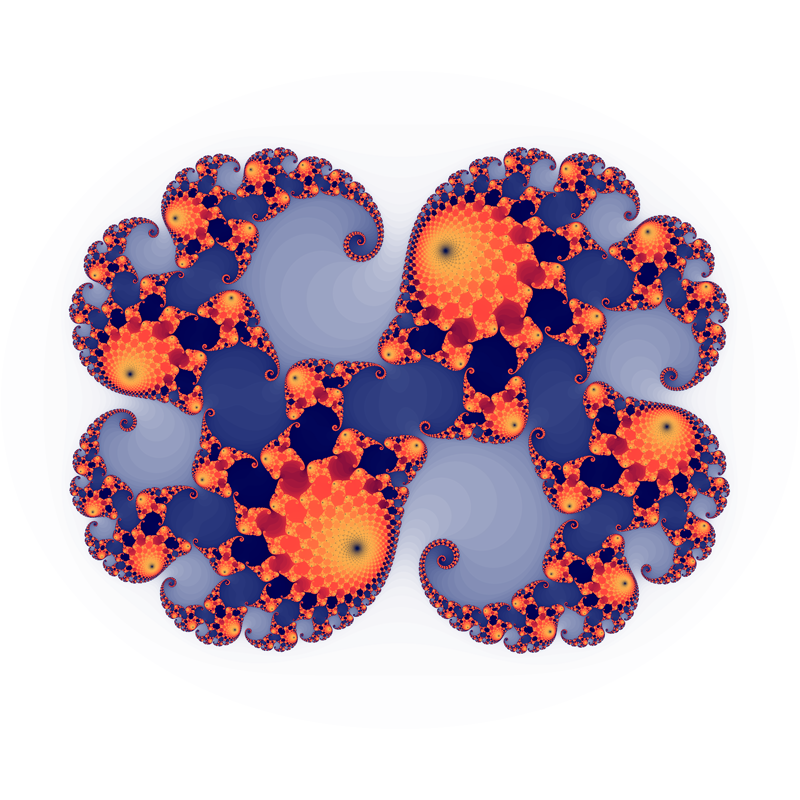c = 0.4 + 0.4i, k = 0.205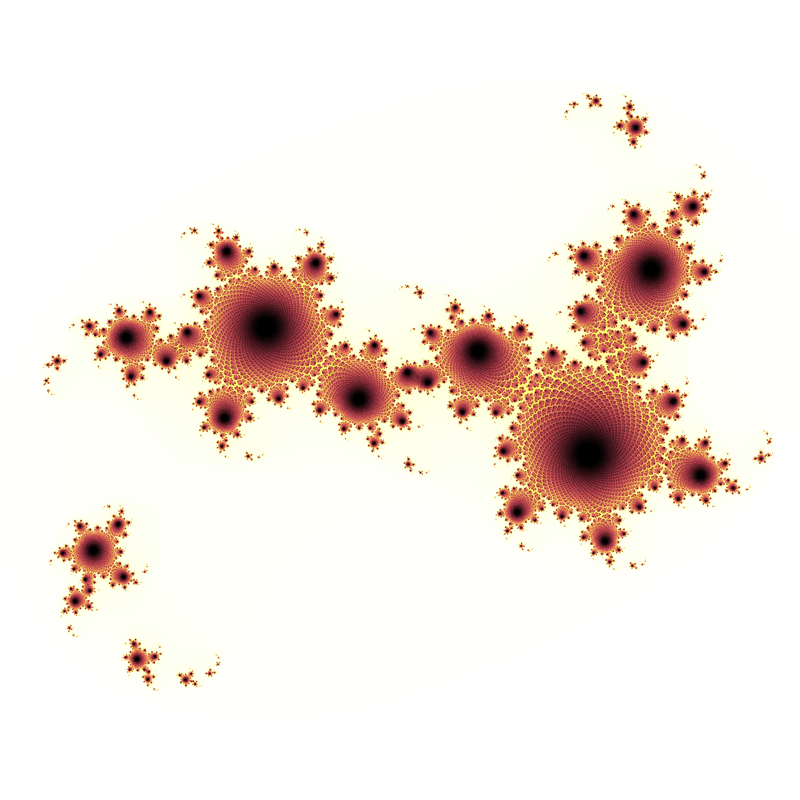c = 0.5i, k = -0.35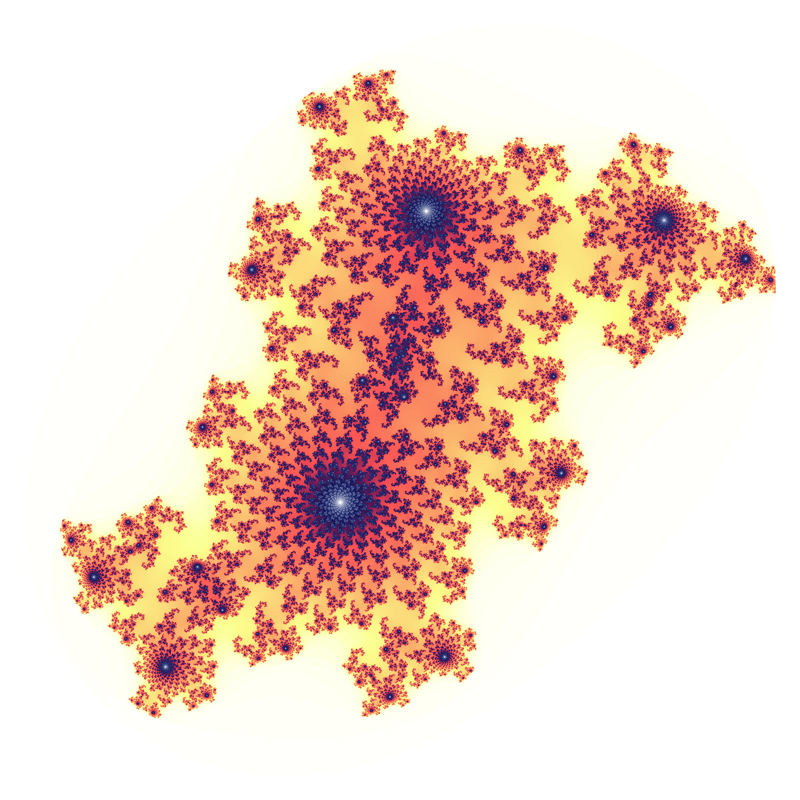c = 0.5i, k = -0.2ic = 0.55 - 0.1ii, k = -0.53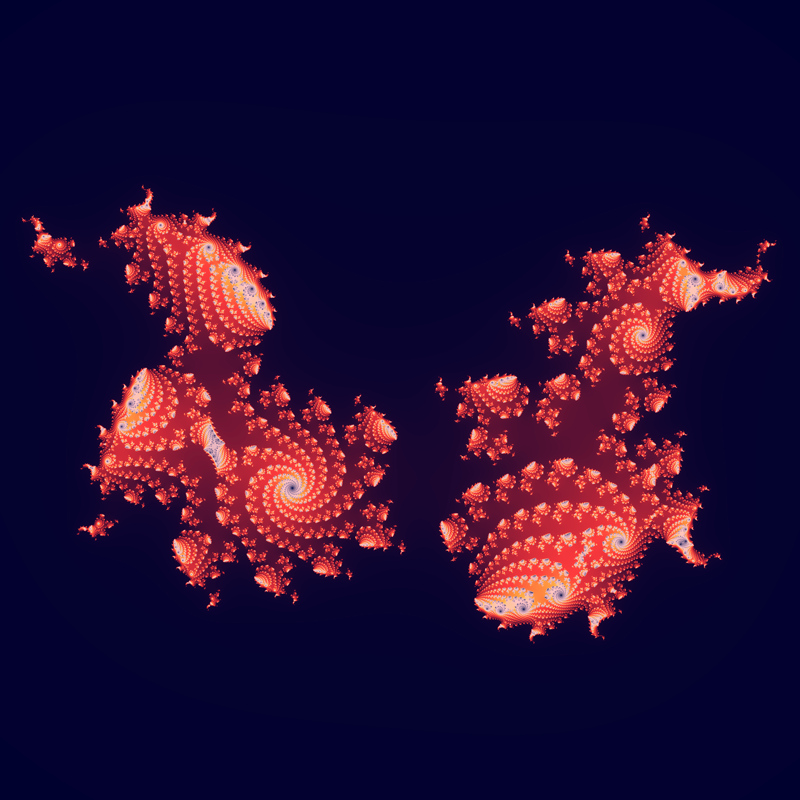c = 0.2 - 0.5i, k = 0.12c = 0.2 + 0.5i, k = 0.1 - 0.2i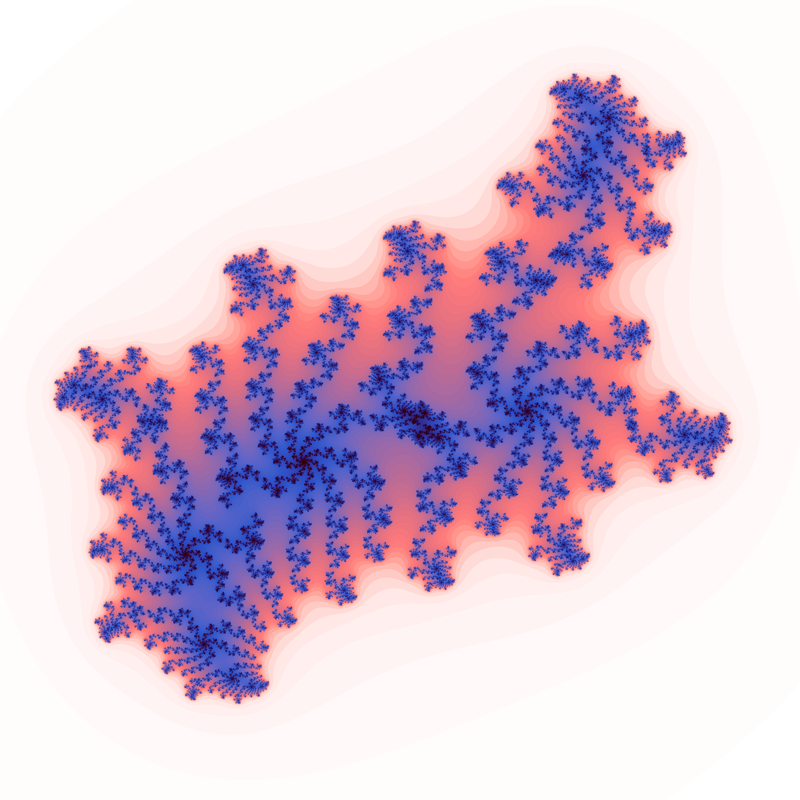c = 0.1 + 0.1i, k = 0.855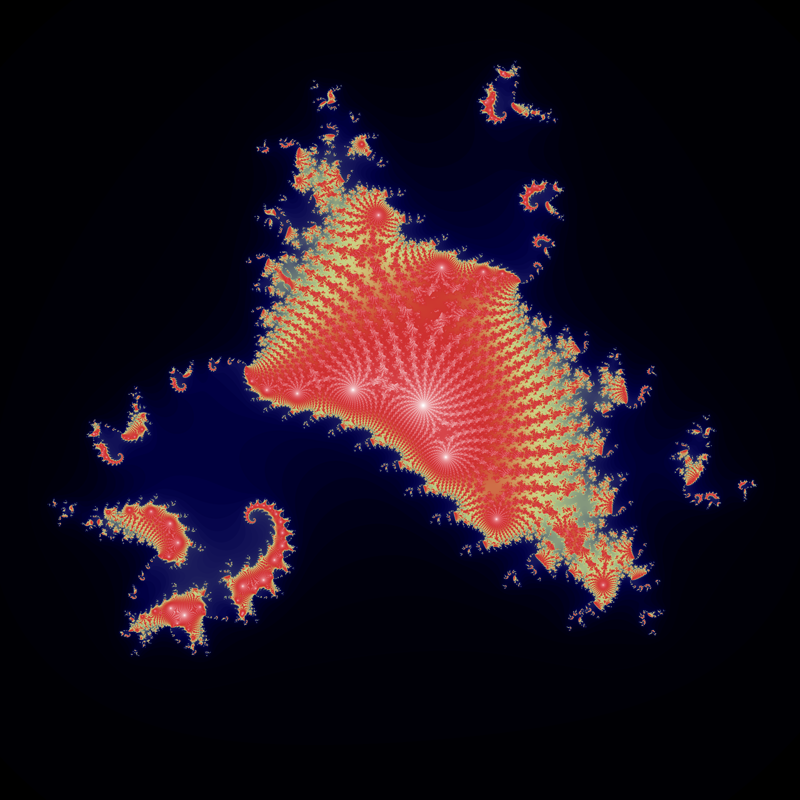c = 0.4 - 0.4i, k = -0.4i